Browse by Topic
Related Topics

# Mathematica Q&A: Four Ways to Sum Integer Digit Blocks

Got questions about Mathematica? The Wolfram Blog has answers! We’ll regularly answer selected questions from users around the web. You can submit your question directly to the Q&A Team using this form.

This week’s question comes from Craig, a hobbyist:

For each six-digit number in a list, how can I check whether the sums of the first and last three digits are equal?

For example, the sums of the first and last three digits of the number 123,222 are equal because 1 + 2 + 3 == 2 + 2 + 2.

There are several different ways of solving this straightforward programming problem in Mathematica, and it’s instructive to compare them. In this post you’ll see four methods demonstrating various combinations of built-in Mathematica functions for working with lists and digits of integers.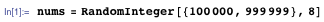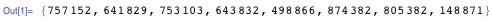Method 1. Using the built-in function IntegerDigits, you can define a handy function to compute the sum of the digits of an integer: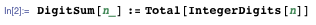To separate the first and last three digits of a six-digit number, you could use the Quotient and the remainder (Mod) when dividing by 1,000: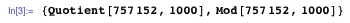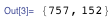Using these two functions with DigitSum, you have the first solution: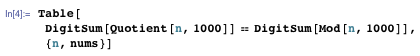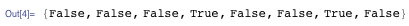Table was used to tabulate the result (in this case, either True or False) for each value of n drawn from the list nums.

Method 2. Getting both the quotient and remainder together is such a common operation that there is a built-in function for it: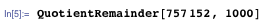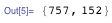If you think about it, this is also the same as finding the base 1,000 digits of a number. You can do this using the two-argument form of IntegerDigits: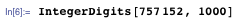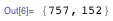Either of these methods of splitting the six-digit number returns a list of two three-digit numbers. To proceed from there, you need to use DigitSum on each element of the list: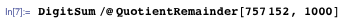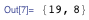The notation f /@ list is shorthand for Map[f, list].

To test whether the elements in a list are equal, you need to use them as arguments to the Equal function. This is exactly what the built-in function Apply is for: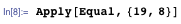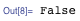Apply[f, list] uses list as the arguments to the function f. In this example it returns Equal[19,8], which evaluates to False.

Putting it all together, here is the second solution: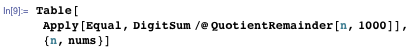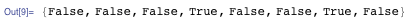Method 3. Instead of splitting the number into two three-digit numbers and using DigitSum, you could begin by getting all six digits as a list and then using Partition to break it into sublists of length three: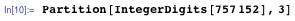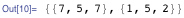You can use Total on each sublist: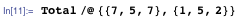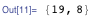Now you can apply Equal as before to get the third solution: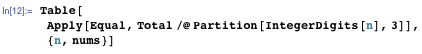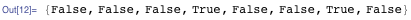Method 4. So far Table has always been used to operate over the list nums. (You also could have used Map for that.) A different approach is to take advantage of the fact that many built-in Mathematica functions automatically operate on lists of arguments, giving a list of results (see Listable).

You can get the QuotientRemainder of all the numbers at once: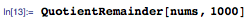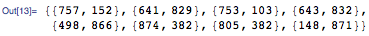Likewise for IntegerDigits (operating on a matrix of numbers and getting a matrix of results):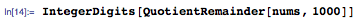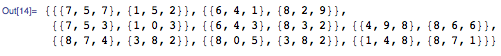The optional second argument of Total totals a nested list specifically at depth three: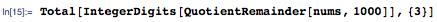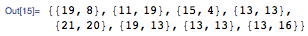Now you can apply Equal to each of these lists of two arguments. There is a special built-in notation, @@@, for this common operation. This yields the fourth solution: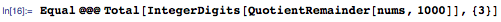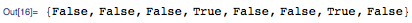(The @@@ is shorthand for Apply[f, expr, {1}], which means “Apply f to each item at depth 1 in expr.”)

Method 4 is the most concise solution in this post. Can you find a shorter one? How about any fundamentally different approaches?

If you have a question you’d like answered in this blog, you can submit it to the Q&A Team using this form.